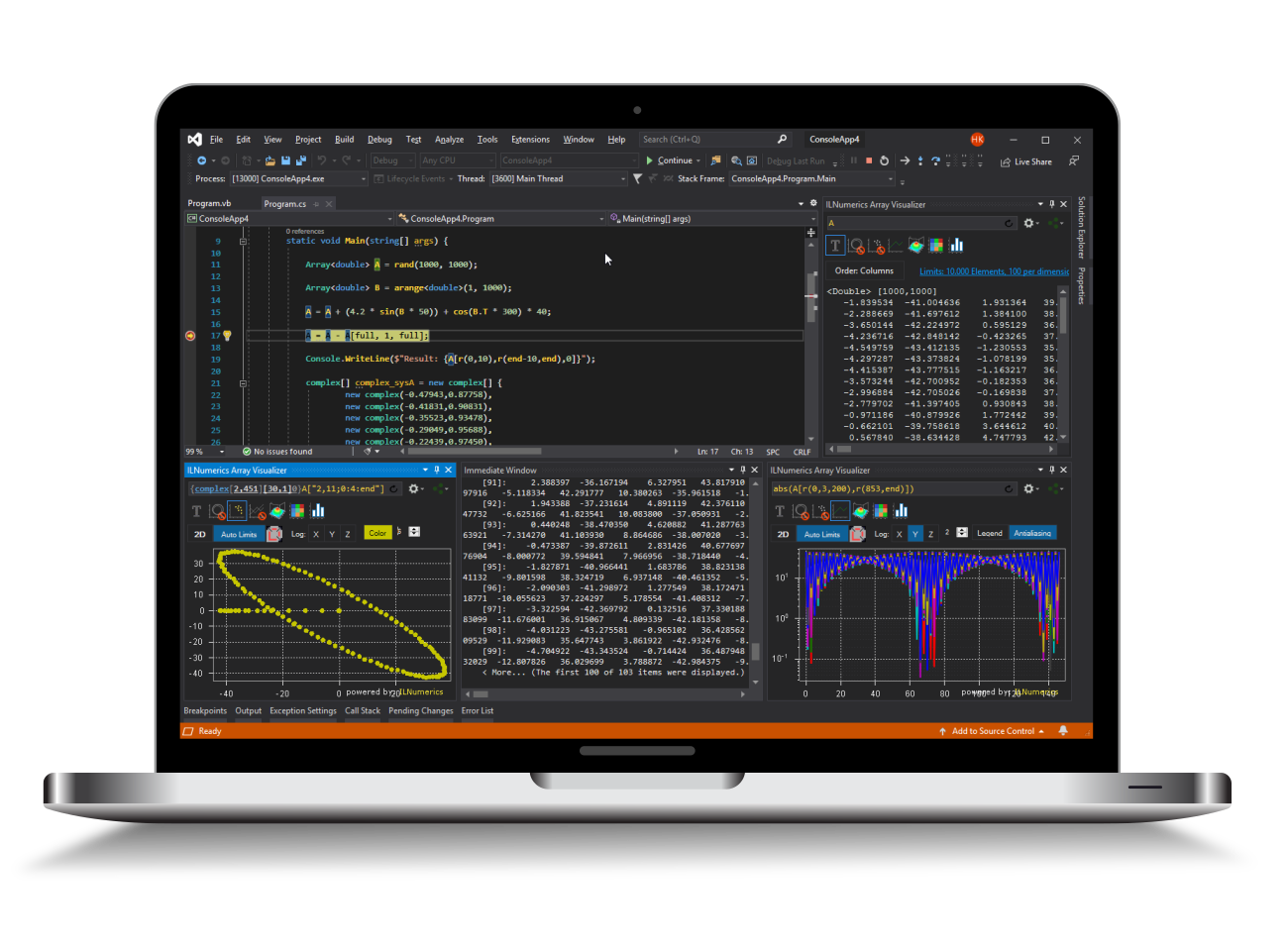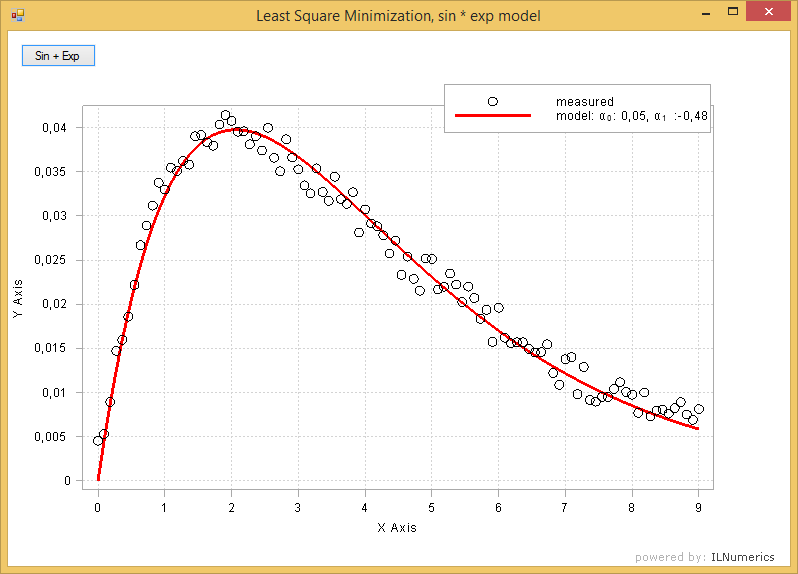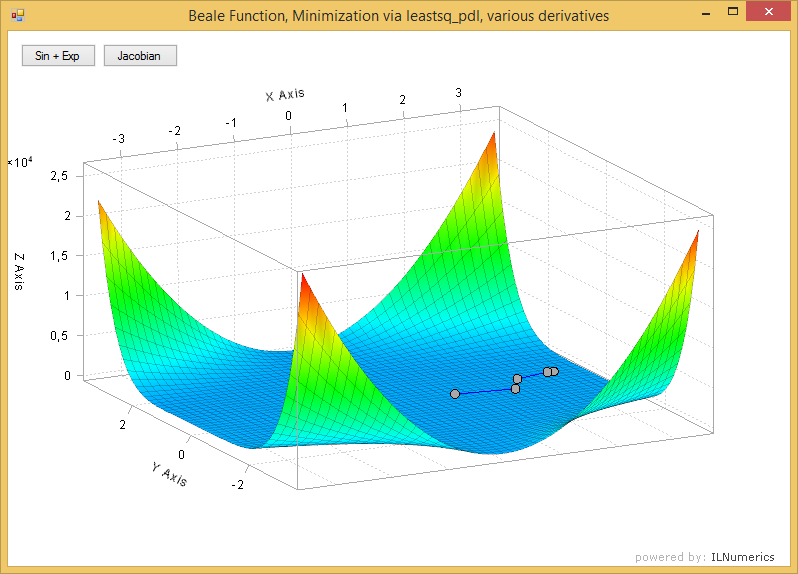Industrial Data Science
in C# and .NET:
Simple. Fast. Reliable.ILNumerics - Technical Computing

Modern High Performance Tools for Technical

Computing and Visualization in Industry and Science

tgt

# Nonlinear Least Squares in .NET (C# and Visual Basic)

Consider having a set of measurement data $\vec y$. The underlying process which generated the data is not (or not completely) known. Therefore, we seek to fit a model function $f(\vec x,\vec\alpha)$ which can be used to model the data generation instead. Such function is useful in order to predict new data values, for visualization, analytics and other purposes.

Least squares optimization aims to optimize the model parameters $\vec\alpha$ to make the model best fit the underlying data generation process. By 'best fit' the one is considered which minimizes the 'error', i.e. the differences between the obtained data $\vec y$ and the data generated by the model $f$ in a least square sense: $$\min_\alpha \|\underbrace{\vec y - f(\vec \alpha,\vec x)}_{F(\vec \alpha)}\|_2^2$$

$$F: \mathbb{R}^m \rightarrow \mathbb{R}^n,\vec\alpha\in\mathbb{R}^m$$

In ILNumerics.Optimization Toolbox this approach is generalized by trying to zero the function $F(\vec \alpha)$ in an iterative process. In the implementation of $F$ arbitrary data, say $\vec y$ or $\vec t$ can be taken into account.

## Nonlinear Programming Algorithms for Least Squares

Two popular algorithms are implemented in ILNumerics Optimization Toolbox:

• Optimization.leastsq_levm - Levenberg-Marquardt (LM) nonlinear least squares solver. Use this for small or simple problems (for example all quadratic problems) since this implementation allows smallest execution times by enabling access to highly optimized objective functions.
• Optimization.leastsq_pdl - Powell's Dog Leg (PDL) algorithm is specialized to more complex problems and those, where the initial guess is far from the optimium. It tends to find the solution in fewer iterations than the LM algorithm in such situations.

### Example

Measurement data $\vec y$ have been acquired by taking samples $\vec y = y_0,y_1,...y_n$ of some system state for roughly every 10ms. The plot of the data shows a damped sinusoidal behavior:

We model the system by $$f(\vec \alpha, t) = sin(\alpha_0 t) e^{\alpha_1 t}$$

Hence, since we want the difference to our real measurement data, our objective function $F$ reads:

$$F(\vec \alpha, t, \vec y) = \vec y - sin(\alpha_0 t) e^{\alpha_1 t}$$

In order to find the parameters $\alpha$ of the model we implement the objective function inline using a lambda expression:

Note that all objective functions must follow the signature of ILNumerics.Optimization.ObjectiveFunction<double>:

that is, the function takes the vector $\vec \alpha$ of length $m$ and returns the vector $F$ of length n, taking the ILNumerics function rules into account. Often, $F$ requires more parameters. Read below for efficient options to deal with that.

Back to our example: the hidden parameters $\alpha_0$ and $\alpha_1$ are found by Optimization.leastsq_levm. We give a starting guess for $\vec \alpha$ of [0.1, 0.1]:

This finds a minimizer $min$ at [0.05; -0.48]. Plotting the model against the original data gives:The full example is found in the examples section.

## Providing additional Data for Least Squares

Most objective functions require more information than $\vec \alpha$, the model parameter to be optimized. In the example above, two additional data vectors are required in order to compute the return value of $F$: the original data as well as the times these data were acquired at. However, the signature of Optimization.ObjectiveFunction<T> is fixed and limited to one input parameter. Moreover, that single parameter holds the complete model parameter set and is varied and provided be the algorithm automatically. How can we bring in the additional data into the implementation of $F$ than?

Two options are available:

1. Lambda expressions. In our example we have used a lambda expression in order to capture the values of $\vec t$ and $\vec y$ and make them available within the objective function. This is the easiest approach and recommended for small setups since it can be implemented almost 'on the fly' by simply referring to any value available at the time of writing the lambda. Keep in mind, even changes to the values captured by the lambda will be visible in the function!
2. Class methods. For more complex situations it is recommended to implement the objective function as instance method of a helper class. Give the class attribuites or properties for all additional parameters needed by the objective function. These attributes are naturally accessible from within the objective function. See here for some hints on using Array<T> as attributes in a class context.

Both options basically do the same thing: capturing additional parameters in a helper class. In the case of lambda expressions, the class is created automatically by the compiler. This releases the user from some boilerplate code writing. On the other hand, writing the class manually gives more flexibility in providing and maintaining the state of those additional parameters and controlling their livetime.

## Controlling the Optimization of Least Squares

The process of finding the solution can be controlled by the user in various ways. Similar options exist as for scalar function minimization: controlling the derivative computation and the exit conditions.

### Jacobian Computation

Both algorithms available require the repeated computation of the first derivative of $F$, the Jacobian matrix $J_F$:

\begin{aligned} J_F \,&= \frac{\partial(F_1\ldots F_n}{\partial(\alpha_1\ldots\alpha_m)} \\ \\ &= \begin{vmatrix} \frac{\partial F_1}{\partial\alpha_1} & \frac{\partial F_1}{\partial\alpha_2} & \ldots & \frac{\partial F_1}{\partial\alpha_m} \\ \vdots & \vdots & \ddots & \vdots \\ \frac{\partial F_n}{\partial\alpha_1} & \frac{\partial F_n}{\partial\alpha_2} & \ldots & \frac{\partial F_n}{\partial\alpha_m} \end{vmatrix} \end{aligned}

Two predefined methods exist in ILNumerics Optimization Toolbox for numerical jacobian computation:

• ILNumerics.Optimization.jacobian_prec - precise estimate of the derivative. This function uses a forward and backward finite differences approach with automatic step size tuning and Ridders' method of polynomial extrapolation. This will give very accurate results and usually leads to much fewer steps to the final solution. However, computing accurate estimates is rather expensive and potentially lead to poor performance. jacobian_prec is the default for Optimization.leastsq_pdl.
• ILNumerics.Optimization.jacobian_fast - estimation of the derivatives with orientation towards speed rather than accuracy. This function uses a forward finite differences approach, a simple stepsize computation (with a fallback to the more advanced algorithm used in jacobian_prec). This leads to fewer evaluations of the objective function to the cost of less accurate results. One may notice a great speed-up in comparison to jacobian_prec for large scale functions. However, the reduced accuracy may or may not lead to more iteration steps to the final solution. This function is recommended for "good behaving" objective functions, with relatively few variables and is the default for Optimization.leastsq_levm.

In order to specify the jacobian computation function to be used for the least square optimization, the jacobianFunc parameter is used:

### Custom Jacobian Functions

Next to the numerical evaluation of the Jacobian, users can provide own implementations for the Jacobian. Here, the Jacobian can be explicitly (analytically) implemented or a custom numerical evaluation can be used.

All derivative functions have the same signature:

Next to the current position $vec\alpha$, the objective function $F$ and the evaluation of the objective function $fx$ is provided. It is up to the implementor which one of the provided parameters to use. Choose those parameters which are helpful for your implementation. If, say, an analytical jacobian definition is known, neither $func$ nor $fx$ are needed. Otherwise, you may utilize these parameters in order to derive a numerical estimate of the Jacobian matrix.

#### Example

The Beale function serves as another popular example in optimization:

$$F_{Beale} = (1.5 - x_1 + x_1x_2)^2 + (2.25 - x_1 + x_1x_2^2)^2 + (2.625 - x_1 + x_1x_2^3)^2$$

Its global minimum $\vec x_{min}$ is known to be found at [3; 0.5] with $F(\vec x_{min}) = 0$:We can use one of the least squares optimization methods in order to find the minimum computationally. The Beale function adds three squared terms. This nicely corresponds to the "sum of squares" expected by our leastsq_??? methods. If we can zero each term seperately, we have zeroed the whole function and found the minimium. Therefore, we write the Beale function in a vectorized form. We also remove the squares, since the individual terms are squared by the least squared algorithm anyways:

$$F_{Beale,vect} = \left[\begin{matrix} 1.5 - x_1 + x_1x_2 \\ 2.25 - x_1 + x_1x_2^2 \\ 2.625 - x_1 + x_1x_2^3 \end{matrix}\right]$$

An implementation might be given by:

The Beale function shows a very flat shape which is challenging for the minimization. (The Levenberg-Marquardt algorithm implemented in leastsq_levm would not be able to find that minimum!) The Powell dogleg method solves that in only 8 iteration steps. However, even for leastsq_pdl subtle differences exist regarding the algorithm performance. Let's compare the number of iterations needed for various derivatives! We compare between the predefined derivative functions and the following custom jacobian function:

Function evaluations needed when using the default Jacobian, the faster variant and the exact Jacobian:

Unsurprisingly, the accurate Jacobian estimate takes the most function evaluations. But even the fast variant is not able to catch up with the exact custom implementation. Take this home: if possible and as soon as either precision or speed matters - the effort of investing into a good custom derivative implementation mostly pays off.

### Exit Conditions

Exit conditions specify when to exit the iteration loop. All parameters are optional and have sensible default values defined.

• maxIter - defines the maximum number of iterations spent inside the optimization loop. Default: 200
• tol - causes the iterations to exit when the current solution is 'close enough' to the optimal solution. Since the exact solution is not known, tol gives a threshold on the displacement of the intermediate solution at each iteration. If the current solution did not move further than tol, the problem is considered as having 'converged'. Default: 1e-8.
• tolf - states a threshold on the function value at the solution which is to be considered an acceptable error. Default: 1e-8.

## Acquiring Convergence Details

Following optional parameters provide more insight into the convergence properties of the algorithm. Their application is identical to the case of unconstrained scalar functions. Please visit the corresponding section there.

• iterationCount – if not null, gives the number of major iterations steps needed to find the solution.
• iterations – if not null, returns intermediate solution vectors as columns of a matrix. The first column holds the initial guess, the last column corresponds to the return value (i.e.: the minimizer of $F$).
• gradientNorms – if not null, returns the norm of the gradients of $F$ at intermediate iteration steps as a row vector. The first element holds the norm of the gradient at the initial guess, the last column corresponds to the minimizer given as return value.

Read more in the section of unconstrained scalar optimization.

## References

Virtual Library of Simulation Experiments - Test Functions and Datasets, http://www.sfu.ca/~ssurjano/optimization.html

D. Marquardt, "An Algorithm for Least-Squares Estimation of Nonlinear Parameters", SIAM Journal of applied Mathematics, Vol. 11, pp. 431–441, 1963

K. Levenberg, "A method for the solution of certain nonlinear problems in least squares", Quart. Appl. Math, pp.164–168, 1944

M.J.D. Powell, "A new algorithm for unconstrained optimization", In: J. Rosen, O. Mangasarian, and K. Ritter, editors, "Nonlinear Programming", pp. 31–65, Academic Press, 1970